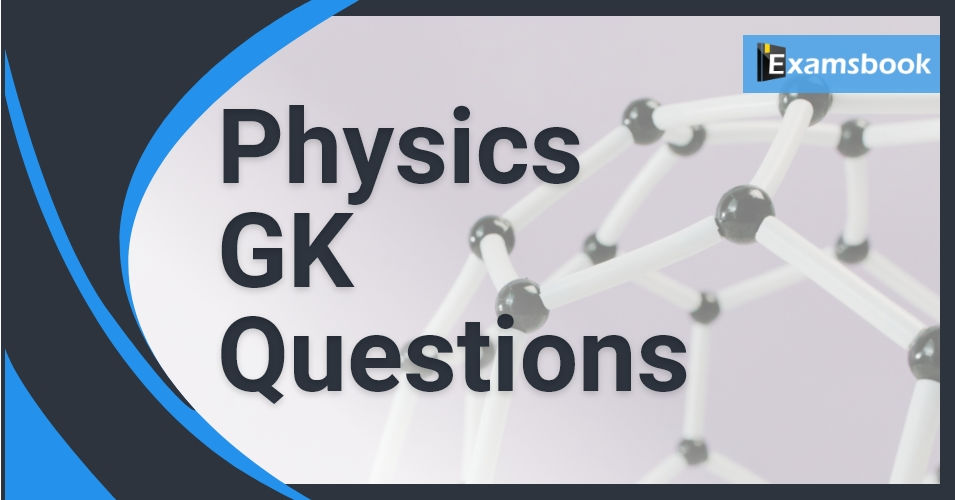• Save

# Physics General Knowledge Questions: Physics GK Question for Competitive ExamsIn this post, I am sharing important physics questions. Practice physics gk with these important and selective physics questions and answers. You should try to memorize this collection of physics general knowledge questions for better results in competitive exams.

If you want to read Gk 2018 at a single page: Visit Gk Questions Blog.

These Physics related questions are useful and there is a strong chance to ask these physics questions again for the competitive exams of 2018 like IAS, Bank PO, SSC CGL, RAS, CDS, UPSC exams, and all state-related exams.

You should also check General Science Questions and Chemistry GK Questions for better practice of Physics General knowledge for Competitive Exams. Try to solve these basic physics questions for better results in SSC.

Physics GK Questions

Q :

When passing through a prism under deflection, which color has the maximum deviation of light?

(A) Red color

(B) Green color

(C) Blue color

(D) violet

Explanation :
violet
In the dispersion of light through a glass prism, violet has the maximum deviation.

Q :

If the reflection formed in the mirror is always perpendicular to the object in shape, is the mirror?

(A) concave

(B) convex

(C) level

(D) None of these

Explanation :

When the object moves with speed u towards (or away) from the plane mirror then image also moves toward (or away) with speed u. But relative speed of image w.r.t. object is 2u.

Q :

What is the approximate time difference between an actual sunset and a virtual sunset?

(A) 2 minutes

(B) 1 minute

(C) 4 minutes

(D) 3 minutes

Explanation :

As a result, the Sun appears to be raised above the horizon and we are able to see the Sun 2 minutes before the actual sunrise. Same phenomenon occurs during sunset, i.e., we can see the Sun 2 minutes after the actual sunset. Thus, the time difference between actual sunset and the apparent sunset is about 2 minutes.

Q :

Which are the two important parts of human beings in which magnetic field must be generated?

(A) arms and legs

(B) muscles and heart

(C) Heart and brain

(D) Eye and visual nerve

Explanation :

Two main organs in the human body where the magnetic field produced is significant, are the heart and the brain. The magnetic field inside the body forms the basis of obtaining the images of different body parts.

Q :

Which physical quantity is obtained from the ratio of momentum and velocity of matter?

(A) Velocity

(B) mass

(C) Angular velocity

(D) Acceleration

Explanation :

The correct answer is Mass. Mass is a quantitative measure of inertia, a fundamental property of all matter. It is the resistance that a body of matter offers to a change in its speed or position upon the application of a force. Mass is represented by the ratio of momentum and velocity of the body.

Q :

What is the force due to which the body is pulled towards the center of the earth?

(A) mass

(B) impulsive force

(C) Gravity

(D) momentum

Explanation :

Everything is pulled towards center of the Earth because of the force of gravity. Gravity is an invisible pull which attracts the objects towards the center of a planet.

Q :

Ice is floating on water in a beaker when the ice melts completely, the level of water in the beaker will be?

(A) will increase

(B) first increase then decrease

(C) will decrease

(D) will remain the same

Explanation :

Therefore, when the ice melts its volume decreases but the volume of water will remain the same. Hence even after the ice melts, there will be no rise in the level of the water. So, the level of the water will remain unchanged.

Q :

Light year is a unit of which of the following physical sign?

(A) Short time interval

(B) mass

(C) Work

(D) distance

Explanation :

Light-year is the unit of length to measure large distances (astronomical distances).

The total distance travelled by light in 1 year is called 1 light year.

1 light-year = 9.46 × 1012 km.

Explanation for the incorrect options

The second (sec) is the unit of time.

A kg is the unit of mass.

Therefore, the Light year is the unit of distance. So, option (D) is correct.

Q :

According to Dalton’s atomic theory, atoms combine in the ratio of small whole numbers to form ______.

(A) Neutrons

(B) Molecules

(C) Protons

(D) Compounds

Explanation :

1. According to Dalton's atomic theory, atoms combine in small whole-number proportions to form compounds.

Q :

Match the columns.

Column - A                         Column-B

I. Electric current               a. Henry

III. Gapacitance                 c. Volt

IV. inductance                    d. Ampere

(A) i-a, ii-b, iii-c, iv-d

(B) i-b, ii-a, iii-c, iv-d

(C) i-d, ii-c, iii-b, iv-a

(D) i-a, ii-c, iii-b, iv-d

Explanation :

The columns are a correct match.

I. Electric current            - Ampere

II. Potential difference    -   c. Volt

IV. inductance                 -   a. Henryd.